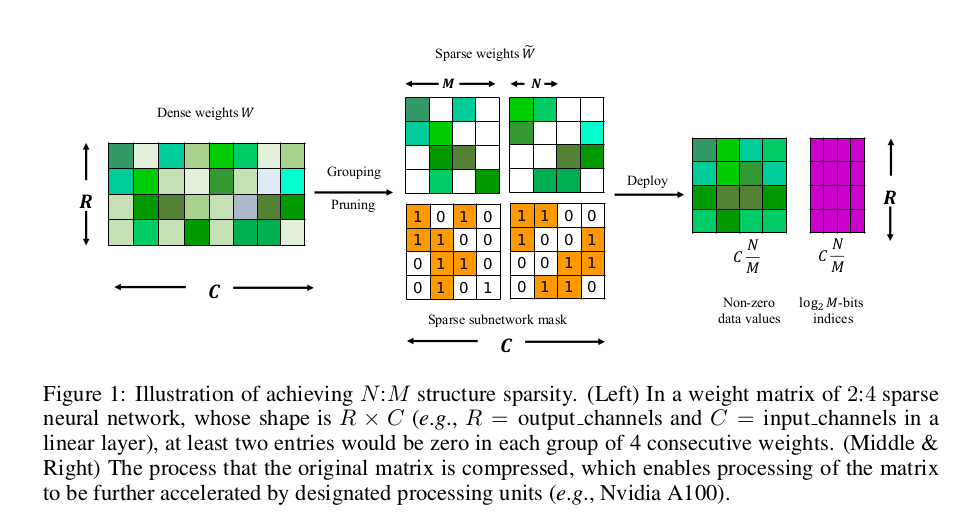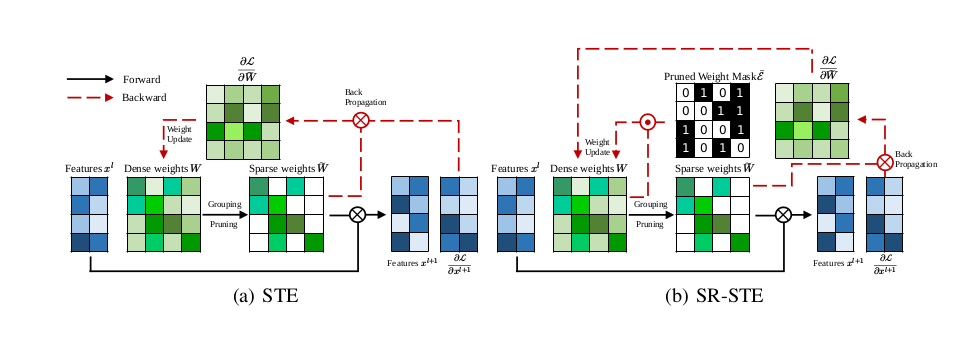Geek Repo

Github PK Tool

# N:M Fine-grained Structured Sparse Neural Networks

## Why N:M sparsity?

Sparse Networks is divided into structured sparsity and unstructured sparsity. Unstructured sparsity can remove network parameters at any position, which is called fine-grained sparsity. Unstructured sparseness can often achieve a higher sparsity ratio and maintain the accuracy of the model, but it is difficult to achieve speedup.

N:M sparsity is fine-grained structured network, which can maintain the advantages of both unstructured fine-grained sparsity and structured coarse-grained sparsity simultaneously.

Thus, latest NVIDIA Ampere design for 2:4 sparsity, this paper discuss a more general form of N:M sparse networks.For hardware acceleration, you can see the following resources:

## Method

SR-STE can achieve comparable or even better results with negligible extra training cost and only a single easy-to-tune hyperparameter $\lambda_w$ than original dense models.the implementation details are shown as follows(in https://github.com/NM-sparsity/NM-sparsity/blob/main/devkit/sparse_ops/sparse_ops.py):

class Sparse(autograd.Function):
"""" Prune the unimprotant weight for the forwards phase but pass the gradient to dense weight using SR-STE in the backwards phase"""

@staticmethod
def forward(ctx, weight, N, M, decay = 0.0002):
ctx.save_for_backward(weight)

output = weight.clone()
length = weight.numel()
group = int(length/M)

weight_temp = weight.detach().abs().reshape(group, M)
index = torch.argsort(weight_temp, dim=1)[:, :int(M-N)]

w_b = torch.ones(weight_temp.shape, device=weight_temp.device)
w_b = w_b.scatter_(dim=1, index=index, value=0).reshape(weight.shape)
ctx.decay = decay

return output*w_b

@staticmethod

weight, = ctx.saved_tensors
return grad_output + ctx.decay * (1-ctx.mask) * weight, None, None

## Experiments

classification

detection

segmentation

language model

#### Citing

If you find NM-sparsity and SR-STE useful in your research, please consider citing:

    @inproceedings{zhou2021,
title={Learning N:M Fine-grained Structured Sparse Neural Networks From Scratch},
author={Aojun Zhou, Yukun Ma, Junnan Zhu, Jianbo Liu, Zhijie Zhang, Kun Yuan, Wenxiu Sun, Hongsheng Li},
booktitle={International Conference on Learning Representations},
year={2021},
}